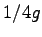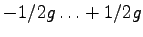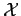## 2. 2. 1 Numerical Discretization Error

In most cases floating point data types are used which have the drawback that each single operation imposes a numerical error. Under some circumstances such a behavior can result in an accumulation or in an extinction of numerical errors. An introduction into the effects of numerical calculations and the difficulties can be found in .

There exist data types which try to imitate the behavior of rational numbers , they generally represent the numerator and the denominator of a fraction and use adaptive integer numbers. By the determination of the greatest common divisor, for instance by the Euclidian algorithm, a cancellation can be performed and the adaptive integers can be kept small.

Even though these calculations are possible in principle, adaptive integer arithmetic operations are slow compared to the highly optimized floating point operations. Moreover, it has to be stated that the closedness of the operation is limited to the memory consumption. There are some numbers, whose sum or product can not be stored due to memory limitations.

In contrast, computational data types typically used are not dense and do have some given granularity. The granularity is the size of an interval in which no number of the given data type is located. When using floating point arithmetic numbers, this interval also depends on the size of the numbers within which the interval is searched.

The numerical error which is made by choosing a discrete representation of a number instead of the ''real'' number can be estimated by, where the error is equally distributed from. For each operation which does not exactly lead to a value within the setof data type numbers, an approximation within the sethas to be determiend.

Michael 2008-01-16